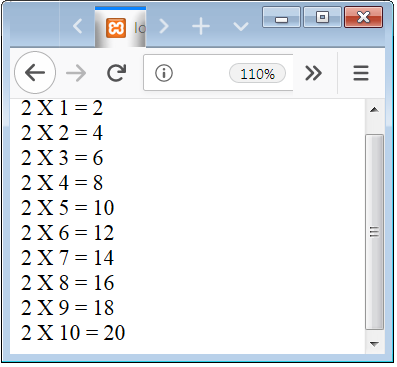# PHP For Loop Statement with Break, Continue Syntax Example Code

## PHP for Loop

Loops are used to execute a part of code multiple times. Loop is also called iterative statements. PHP supports 4 type of loops such are “for loop”, “while loop”, “do-while loop”,& “foreach loop”.

for Loops are used to execute a part of code multiple times. In for Loop initialization, condition and increment/decrement all these three statements are declared fist.

### for Loop Syntax

```<!DOCTYPE html>
<html>

<body>
<?php
for(initialization; condition; increment/decrement)
{
code to be executed;
}
?>
</body>
</html>
```

### Example 1 – for Loop Example Code to print Series of numbers

```<!DOCTYPE html>
<html>

<body>
<?php

for(\$x=1; \$x<=5; \$x++)
{
echo \$x ."<br />";
}

?>
</body>
</html>
```

In the above code, we describe how you can use syntax of for Loop.

Here we declare a variable \$x of value 1 and condition is that the value of \$x is less than or equal to 5 and increment of the variable \$x by one and echo the value of \$x.

## Print the Table of 2 using for Syntax

The bellow code is used to print the table of 2 with use of for loop.

### Example 2 – Print the Table of 2 using for, example to print the series 2,4,6—10.

```<!DOCTYPE html>
<html>

<body>
<?php

for(\$x=1; \$x<=10; \$x++)
{
echo "2 X " . \$x . " = " . \$x * 2 . "<br />";
}

?>
</body>
</html>
```

In the above code, we describe how you can use syntax of for Loop and print the table of 2.Fig.2 – for Loop Example to Print Table of 2.

Note: Similarly, you can create the table of any value you want.

## PHP for Loop Break and Continue Statement Syntax

Break and Continue is used to manipulate the execution of the Loop. Where the Break is used to exit or stop the execution of Loop and Continue is used to skip a part of loop execution.

### Example 3 – PHP for Loop Break Example to exit from the loop in some particular test condition.

```<!DOCTYPE html>
<html>

<body>
<?php

for(\$x=1; \$x<=10; \$x++)
{
if(\$x==5)
{
break;
}
echo "2 X " . \$x . " = " . \$x * 2 . "<br />";
}

?>
</body>
</html>
```

In the above code, we describe how you can use syntax of for Loop and print the table of 2.

Here we break the loop when the value of \$x is 5.

### Example 4 – PHP for Loop Continue Example to skip execution of some part of code in some test condition.

```<!DOCTYPE html>
<html>

<body>
<?php

for(\$x=1; \$x<=10; \$x++)
{
if(\$x==5)
{
Continue;
}
echo "2 X " . \$x . " = " . \$x * 2 . "<br />";
}

?>
</body>
</html>
```

In the above code, we describe how you can use syntax of for Loop and print the table of 2.

Here when the value of \$x is 5 the value of table 2 is skip or not display.